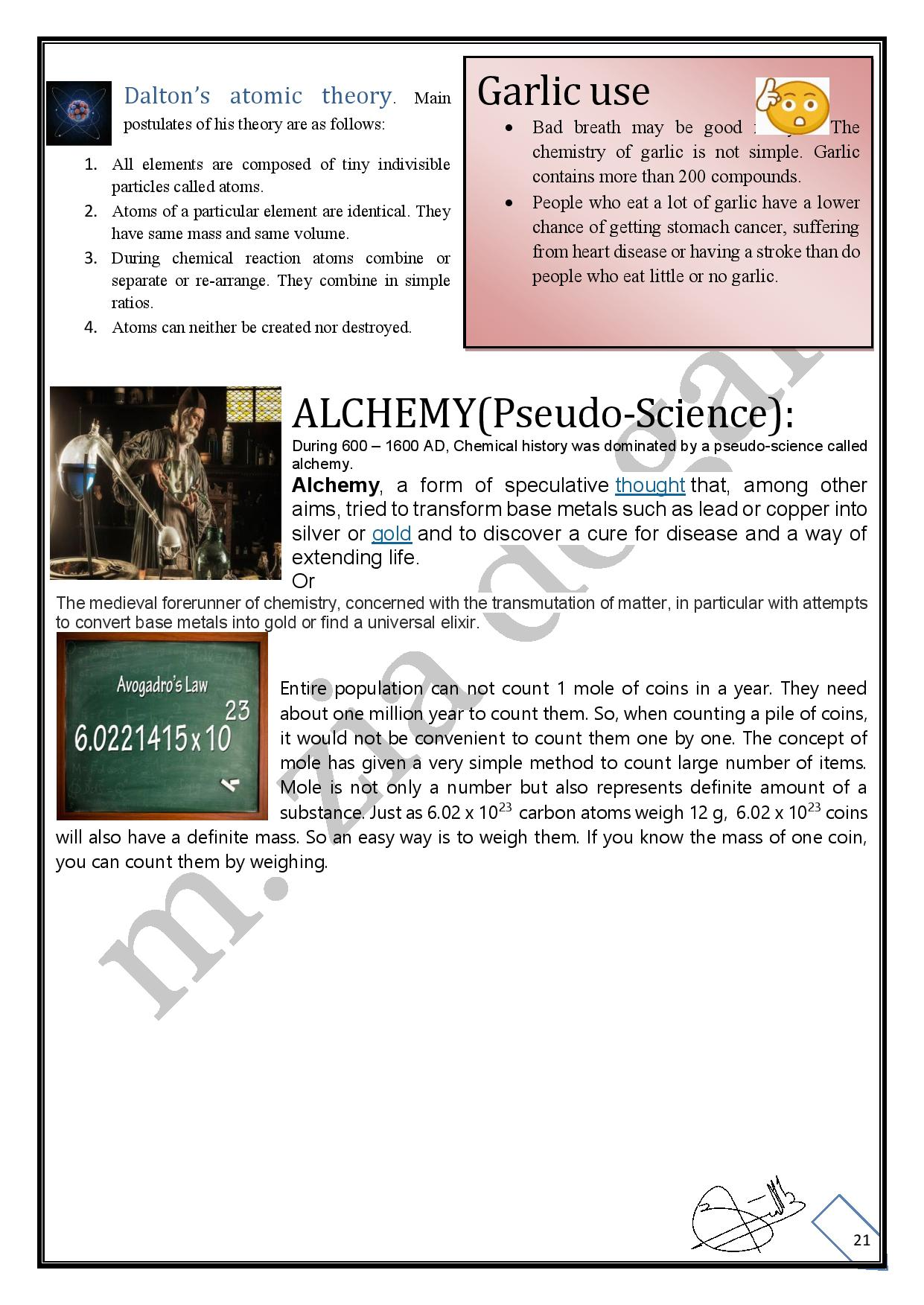# Capter: 01, FUNDAMENTALS OF CHEMISTRY-9th (FBISE Notes)

Fundamentals of Chemistry

Science

It is the knowledge that provides an understanding of this world and how it works.

Chemistry

The branch of science that deals with the composition, structure, properties, and reactions of matter, is called chemistry.

Importance of chemistry in our daily life

1. We live in a world of chemicals.
2. We use many important chemicals daily such as drugs, detergents, paper, paints, pigments, insecticides, pesticides and many petrochemical products.
3. Chemistry provides us knowledge and techniques which are used to improve our health and environment.
1. Chemistry helps us to explore and conserve natural resources.
2. On the other hand, the development of the chemical industry has certain disadvantages also. For example, it has generated toxic wastes, contaminated water and polluted air around us.

HISTORICAL BACKGROUND OF KNOWLEDGE ABOUT MATTER

Greek philosophers

An ancient Greek philosopher, Empedocles thought that all the matter was made up of only four elements:

1) Earth  2) Air      3) Water                4) Fire

The Greek concept of the four elements existed for more than two thousand years.

Dalton’s Atomic Theory

At the beginning of the 19th century, Dalton proposed an atomic theory. According to this theory matter is made up of small indivisible particles called atoms. The atoms of any one element are identical in all respects but different from those of other elements.

Modern picture of an atom

Modern research has clearly shown that the atom is further composed of sub-atomic particles such as electron, proton and neutron.

Q: What are the different branches in chemistry?

Branches of Chemistry

The study of chemistry is divided into the following main branches.

1. Physical Chemistry

It is the study of the Laws and theories that describe the structure and changes of matter.

1. Organic Chemistry

It is the study of covalent compounds of carbon and hydrogen (hydrocarbons) and their derivatives.

1. Inorganic chemistry

It deals with the study of all elements and their compounds except organic compounds.

1. Biochemistry

It is the study of physical and chemical changes that occur in living organisms.

1. Industrial Chemistry

The branch of chemistry that deals with the manufacturing of chemical substances on the commercial scale is called industrial chemistry.

1. Nuclear Chemistry

The study of changes that occur in atomic nuclei is called nuclear chemistry.

1. Environmental Chemistry

It deals with the chemicals and toxic substances that pollute the environment and their adverse effect on human beings.

1. Analytical Chemistry

It deals with the methods and instruments that determine the composition of matter.

Differentiation between branches of Chemistry

Acetic acid chemical formula: CH3 COOH                                        Smell: Vinegar like smell

Source: Vinegar which contains 5% acetic acid.                              Use: Used to flavour food.

Let’s see how various types of studies on this compound can help us to differentiate between the above mentioned eight branches of chemistry.

1. Since acetic acid is a carbon compound, the study of its methods of preparation and its chemical properties is a part of organic chemistry.
2. The study of all chemical reactions that acetic acid undergoes in the bodies of human beings is called biochemistry.
3. The component elements of acetic acid are carbon, hydrogen and oxygen. The study of this element is included in inorganic chemistry.
1. Use of technology and ways to obtain acetic acid on the large scale is done in chemical industries, so it is a part of industrial chemistry.
2. Explanation of conversion of acetic acid from solid to liquid and liquid to gaseousstate and vice versa,
1. The application of laws and theories to understand its structure.

These two types of studies are a part of physical chemistry.

1. The methods and instruments used to determine its percentage composition melting and boiling points etc are studies in analytical chemistry.
2. The study of any adverse effects of the compound or the compounds that are derivedfrom it, on the human is part of environmental chemistry.
3. The study of radioactive radiation or neutron on this compound is a part of nuclearchemistry.

Identifying examples of different branches of chemistry

1. Photosynthesis produces glucose and oxygen from carbon dioxide and water in presence of chlorophyll and sunlight.
2. Plantation helps in overcoming green house effect.
• Haber’s process converts large quantities of hydrogen and nitrogen into ammonia (NH3).
1. Ammonia is a colourless gas with pungent irritating odour. It is highly soluble in water.
2. A chemist performed an experiment to check the percentage purity of a sample of glucose (C6H1206).
3. An analyst determines that N02 is responsible for acid rain.
• Chlorofluorocarbon compounds are responsible for the depletion of ozone layer.
• α-particles (He++) when bombard on nitrogen atom, a proton is emitted.

Solution

1. Biochemistry, since photosynthesis is a chemical reaction that occurs in plants (living organism).
2. Environmental chemistry, since green house effect is an environmental problem.
1. Industrial chemistry, since large scale production of any substance is the subject of industrial chemistry.
1. Inorganic chemistry, since it deals with properties of inorganic compounds.
1. Analytical chemistry, since it deals with analysis of a compound, whether organic or inorganic in nature.
2. Environmental chemistry, since acid rain is an environmental problem.
3. Environmental chemistry, since depletion of ozone layer is an environmental problem.
4. Nuclear chemistry, since nuclear change can emit protons.

SELF ASSESSMENT EXERCISE 1.1

Identify the branch of chemistry that is related to the following information:

1. Hair contains a special class of proteins called keratins, which are present in nails and .

1. Acetylene is the simplest hydrocarbon that contains carbon-carbon triple bond. Hydrocarbons are compounds of carbon and hydrogen.

• White lead is a pigment used by artists for centuries, the metal Pb in the compound is extracted from its ore, galena (PbS).

1. Sulphuric acid (H2SO4) is weaker than hydrochloric acid.

1. Gases can be compressed by applying pressure.

1. Some examples of complete protein food are meat, milk and eggs.

• Element radium decays by emitting a-particles and is converted into another element radon.

8) Calorimeter is a device that measures the amount of heat, a substance absorbs on heating or emits on cooling.

BASIC DEFINITIONS

Matter

Anything that has mass and occupies space is called matter.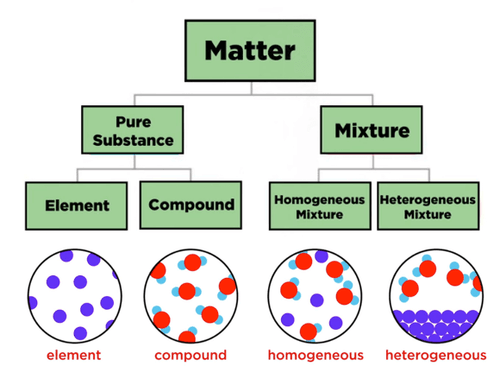Examples: Water, wood, air, paper, gold etc.

Classification of Matter

All materials are either a substance or a mixture. The following figure shows simple classification of the matter into different forms.

Substance: It is a matter that has a particular set of characteristics which differ from the character of any other kind of matter.

Examples

Oxygen, water, urea, glucose and common salt are different substances.

Element

The substance that cannot be converted into other simpler substances is called an element.

Oxygen, hydrogen, Iron, copper and gold are few examples of elements.

Modern definition of Element

It is a substance made up of same type of atoms, having same atomic number.

Compound

A compound is a pure substance that is made up of two or more elements held together in fixed ratios by natural forces called chemical bonds.

The properties of compounds are different from the properties of the elements from which they are formed. Elements and compounds have uniformed composition throughout.

Examples

Water, carbon dioxide, copper sulphate, sodium chloride etc. are compounds.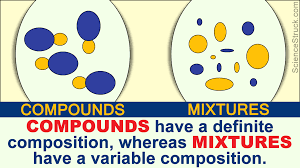Mixture

An impure substance that contains two or more pure substances which retain their individual chemical characteristics is called a mixture.

The entire world is made up of mixture of element and compound. A mixture can be converted into two or more pure substances by a physical method.

Examples

Air, water containing dissolved oxygen, table salt dissolved in water, salt and etc..

Types of Mixture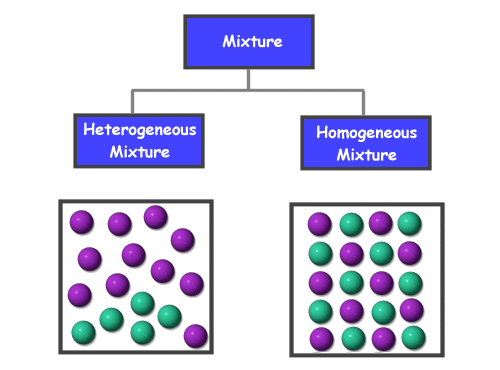1. a) Homogenous Mixture

A mixture that consists of only one phase is called homogenous mixture.

Examples

Salt or sugar dissolved in water, air etc Homogenous mixtures have uniformed composition throughout.

1. b) Heterogeneous Mixture

A mixture that consists of two or more visibly different components is called heterogeneous mixture.

Examples

Sand + Salt, oil floating on water, a class room etc.

DIFFERENCES BETWEEN A COMPOUND AND MIXTURE

The following are important differences between a compound and a mixture

Table Difference between a Compound and a Mixture

 Compound Mixture i. It is formed by a chemical combination of atoms of the elements. Mixture is formed by the simple mixing up of the substances. ii. The constituents lose their identity and  from a new substance having entirely different properties from them. Mixture shows the properties of the constituents. iii. Compounds always have fixed composition by mass. Mixtures do not have fixed composition. iv. The components cannot be separated by physical means. The components can be separated by simple physical methods. ‘ V. Every compound is represented by a chemical formula. It consists of two or more components and does not have any chemical formula. vi. Compounds have homogeneous composition. They may be homogeneous or heterogeneous in composition vii. Compounds have sharp and fixed melting points, Mixture does not have sharp and fixed melting points.

Atomic Number

The number of protons in the nucleus of an atom is known as its atomic number.

It is represented by symbol “Z”. As all atoms of an element have the same number of protons in their nucleus, they have same atomic number.

Examples               All hydrogen atoms have 1 proton, their atomic number is 1. Similarly, all atoms in carbon have 6 protons, their atomic number is 6.

Mass Number

The total number of protons and neutrons is an atom in known as its mass number. It is represented by symbol ‘A’.

Examples

A hydrogen atom has one proton and no neutron in its nucleus hence its mass number is 1. The carbon atom has 6 protons and 6 neutrons; hence its mass number is 12.

The difference between the mass number and the atomic number is equal to the number of neutrons in the nucleus

No of neutron = Mass number – Atomic number = A – Z

Example 1.2

Determining the number of protons and neutrons in an atom.

The atomic number of an element is 17 and mass number is 35. How many protons and neutrons are in the nucleus of an atom of this element?Solution

Number of protons = atomic number = 17

Number of neutrons = mass number – atomic number

= 35-17 = 18

Relative atomic mass

An atom is extremely small particle, therefore we cannot determine the mass of a single atom. However it is possible to determine the mass of one atom of an element relative to another experimentally. The light isotope of carbon (C-12) has been chosen as a standard, with reference to which atomic masses of all other elements can be determined

Thus

“The mass of an atom of an element relative to the mass of an atom of C-12 is called relative atomic mass”

1. Atomic Mass Unit (amu)

One atomic mass unit is one-twelfth the mass of one atom of carbon-12

Mass of one C-12 atom =12 amu

1 amu =

Examples

An atom of Hydrogen is 8.4% as massive as C-12. Therefore relative atomic mass of = 1.008 amu

Similarly, relative atomic masses of oxygen and sodium are 15.9994 amu and 22.9898 amu respectively.

Chemical Formula

Compounds are represented by chemical formulas. A chemical formula tells us:

• Which elements are present in a compound?
• The whole number ratio of their atoms thus a chemical formula shows the type and the number of each atom in a compound.

Types of chemical formulas.

There are several types of chemical formulas but two important types are:

1. Empirical Formula 2. Molecular Formula

Empirical Formula

It gives the simplest whole-number ratio of atoms present in a compound

Examples

The covalent compound hydrogen peroxide has simplest ratio of 1:1 of hydrogen to oxygen. Therefore, its empirical formula is HO. Similarly the simplest ratio between C, H and O atoms in glucose is 1:2:1. Hence its empirical formula is CH2O

Molecular Formula

It gives the actual whole number ratio of atoms of each element present in a molecule of that compound.

Examples

The actual ratio of hydrogen to oxygen atoms in a molecule of hydrogen peroxide is 2:2. Hence its molecular formula is H2O2

The actual ratio between C, H and O atoms in a glucose molecule is 6:12:6, so its molecular formula is C6H12O6

What is the relationship between empirical and molecular formula

We know that molecular formula of a compound may be some as its

empirical formula e.g. H2O CO2, HCl, CH4, CCI4, NH3, HBr, PCl3, H2S04 etc.

Molecular formula may be obtained by multiplying empirical formula with number ‘n’ Molecular Formula = n x Empirical formula = (Empirical formula)n

Where n is 1, 2, 3 and so on.

For example, molecular formula of benzene is C6H6 which is derived from the empirical formula CH where the value of n is 6. The following Table shows some compounds with their empirical and molecular formulas.

The Empirical Formulae of Some of the Compounds

 No. Molecular formula Empirical formula 1. Ethene(C2H6) CH3 2. Ethene (C2H4) CH2 3. Diborane (B2H6) BH3 4. Nitrogen tetraoxide (N2O4) NO2 5. Water (H20) h2o 6. Sodium carbonate (Na2CO3) Na2CO3 7. Hydrogen Peroxide H2O2 HO

SELF ASSESSMENT EXERCISE 1.2

Write the empirical formulas for the compound containing carbon to hydrogen in the following ratios.

1. a) 1:4 b) 2:6     c) 2:2                      d) 6:6

1. a) CH4 b) CH3 c) CH                       d) CH

For many compounds, empirical and molecular formulas are same. For example water (H2O), carbon dioxide (CO2), ammonia (NH3), methane (CH4), sulphur dioxide (SO2) etc. Can you show it why?

The molecules of these compounds contain atoms in their simplest ratios.

SELF ASSESSMENT EXERCISE 1.3

1. Aspirin is used as a mild pain killer. There are nine carbon atoms, eight hydrogen atoms and four oxygen atoms, in this compound. Write its empirical and molecular formulas.

The empirical and molecular formula is same i.e. C9H8 O4

1. Vinegar is 5% acetic acid. This contains 2 carbon atoms, 4 hydrogen atoms and 2 oxygen atoms. Write its empirical and molecular formulas.

Molecular formula: C2H4O2

Empirical Formula: CH2O

1. Caffeine (C8H5N402) is found in tea And coffee. Write the empirical formula for caffeine.

Empirical formula: C4H5N2O

What do you understand by the terms Molecular Mass and Formulamass? How these masses can be determined?

Molecular Mass

It is the sum of atomic masses of all the atoms present in the molecule.

Example

Molecular mass of water (H2O) = 2 (atomic mass of H) + atomic mass oxygen

= 2(1) + 16= 18 amu

PROBLEMS:

1. Determine the molecular mass of glucose, C6H206, which is also known as blood sugar.
2. Determine the molecular mass of naphthalene C10H8, which is used in mothballs.

Solution

Molecular mass of C6H12O6 =          = 6(12.00) + 12(1.008) + 6(16.00)

= 180.096 amu

Molecular mass of Ci0H8                      =12×10+1×8

= 120 + 8 = 128 amu

2) Formula Mass

The term formula mass is used for ionic substances. Ionic compounds consist of oppositely charged ions rather than separate molecules. So we represent an ionic compound by its formula unit.

Formula Unit

It indicates the simplest ratio between cations and anions in an ionic compound.

Example

The common salt consists of Na+ and Cl¯ ions. It has one Na+ ion for every Cl¯ ion.

So formula unit for common salt is NaCl.

Formula Mass

The sum of the atomic masses of all the atoms in the formula unit of a substance is called formula mass.

Examples

The formula mass of sodium chloride is 58.5 amu and that of CaC03 is 100 amu.

How to determine the formula mass?

Example 1.4

Determining formula mass

• Sodium Chloride, also called table salt is used to flavour food, preserve meat, and in the preparation of large number of compounds. Determine its formula mass.
• Milk of magnesia which contains Mg(OH) 2 is used to treat acidity. Determine its formula mass.

Problem-solving strategy

Add the atomic masses of all the atoms in the formula unit.

Solution

Formula mass of NaCl = 1 x Atomic mass of Na +1 x Atomic mass of Cl

= 1 x 23 + 1 x 35.5 = 58.5 amu

Formula mass of Mg (OH) 2 =              24+16×2+1 x 2

=24 + 32 + 2 = 58 amu

1. SELF ASSESSMENT EXERCISE 1.4
2. Potassium Chlorate (KCIO3) is used commonly for the laboratory preparation of 0 Calculate its formula mass.

Formula Mass of KCIO3 = 39 + 35.5 + 3 (16)

= 122.5 amu

1. When baking soda, NaHCO3 is heated it releases carbon dioxide, which is responsible for the rising of cookies and bread. Determine the formula masses of baking soda and carbon dioxide.

Formula Mass ofNaHCO3 = 23 + 1 + 12 + 3(16)

= 84 amu

Formula Mass of CO2         = 12 + 2(16)

= 44 amu

1. Following compounds are used as fertilizers. Determine their formula masses.

Urea, (NH2)2CO, Ammonium nitrate, NH4NO3

Formula Mass of Urea = 2(14) + 4(1) + 12 + 16

= 60 amu

Formula Mass of NH4NO3 = 14 + 4+ 14 + 3 (16)

= 80 amu

Chemical species

Matter is composed of chemical species. These species are atoms, ions, free radicals, molecules and formula units.

Atom

Atom is the smallest particle of an element that cannot exist in Free State it is electrically neutral.

Ion

Ion is an atom or group of atoms having a charge on it.

Types of ions

There are two types of ions.

1) Cations              2) Anions

1) Cations

An atom or group of atoms having positive charge on it is called cation. Metal atoms generally lose one or more electrons and formcations.

Examples

Na form Na+ by losing one electron, Ca forms Ca2+ by losing two electrons.

2) Anions

An atom or group of atoms having negative charge on it is called anion. Non-metals usually gain one are more electrons and form anions.

Examples

Chlorine atom gains one electron and forms Cl¯ ion, O atom gains two electrons and form O2- ion.

why an ion acquires a net positive or negative charge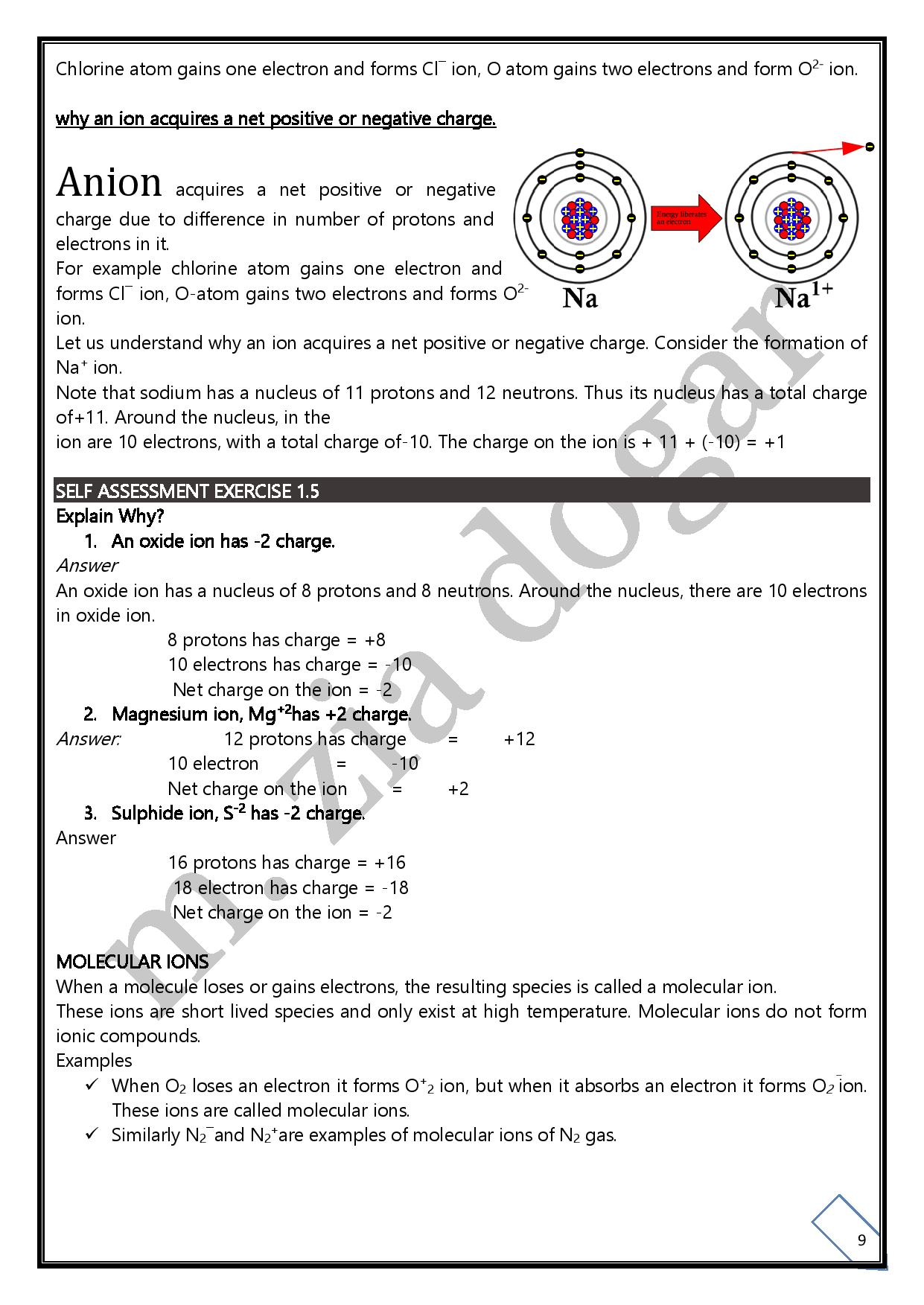Anion acquires a net positive or negative charge due to difference in number of protons and electrons in it.

For example chlorine atom gains one electron and forms Cl¯ ion, O-atom gains two electrons and forms O2- ion.

Let us understand why an ion acquires a net positive or negative charge. Consider the formation ofNa+ ion.

Note that sodium has a nucleus of 11 protons and 12 neutrons. Thus its nucleus has a total charge of+11. Around the nucleus, in the

ion are 10 electrons, with a total charge of-10. The charge on the ion is + 11 + (-10) = +1

SELF ASSESSMENT EXERCISE 1.5

Explain Why?

1. An oxide ion has -2 charge.

An oxide ion has a nucleus of 8 protons and 8 neutrons. Around the nucleus, there are 10 electrons in oxide ion.

8 protons has charge = +8

10 electrons has charge = -10

Net charge on the ion = -2

1. Magnesium ion, Mg+2has +2 charge.

Answer:                  12 protons has charge        =              +12

 No. Ion Free Radical i) An atom or group of atoms having charge An atom or group of atoms having unpaired electrons ii) They exist in solution or in crystal lattice. They exist in solution as well as in air. iii) Ion cannot be produced with the help of light. Light may produce free radicals.

10 electron                           =              -10

Net charge on the ion         =              +2

1. Sulphide ion, S-2 has -2 charge.

16 protons has charge = +16

18 electron has charge = -18

Net charge on the ion = -2

MOLECULAR IONS

When a molecule loses or gains electrons, the resulting species is called a molecular ion.

These ions are short lived species and only exist at high temperature. Molecular ions do not form ionic compounds.

Examples

• When O2 loses an electron it forms O⁺2 ion, but when it absorbs an electron it forms O2¯ These ions are called molecular ions.
• Similarly N2¯and N2⁺are examples of molecular ions of N2

Difference between Molecule and Molecular Ion

 No. Molecule Molecular Ion (i) It is the smallest particle of an element or compound which can exist independently and shows all the properties of that compound. It is formed by gain or loss of electron by a molecule. ii) It is always neutral. It can have negative or positive charge. iii) It is formed by the combination of atoms. It is formed by the ionization of molecule. iv) It is a stable unit. It is reactive specie.

Free radicals are atoms or group of atoms having odd number of (unpaired) electron. Free radicals are electrically neutral species.

Some substances like halogens when exposed to sun light, their molecules split up into free radicals. They are represented by putting a dot over the symbol of an element.

Examples

Cl°, H°, H3C° are examples of free radicals.

Differences between Ion and free radicals

SELF ASSESSMENT EXERCISE 1.6

Identify ions, molecular ions and free radicals from the following species.

CN‾,CN,He+2,  Ӧ, N

1. Ions: N-3
2. Molecular ions: He+2 , CN‾
3. Free radicals: N, O, CN

Representative particles of an element or compound

The representative particles of a substance are atoms, molecules or formula units.

The following example helps us to identify the particles of elements and compounds.

Molecule

Smallest particle of a covalent compound or an element which can exist independently is called molecule.

Types of Molecules Molecules can be classified as:

1. i) Monoatomic ii) Polyatomic

Monatomic

A molecule that contains only one atom is called monoatomic molecule.

Examples

Inert gases consist of monoatomic molecules such as He, Ne, Ar, Kr and Rn.

Polyatomic Molecules

A molecule that contains two or more similar or different atoms called polyatomic is molecule.

Examples

• H2, O2, N2, CI2, S8etc are polyatomic molecules of different elements.
• H2O, HBr, HCI, NH3, H2SO4 etc are polyatomic molecules of different compounds.

Avogardro’s Number

The number of atoms, ions or molecules present in one mole of a substance is called Avogadro’s number. It is denoted by NA and is equal to 6.02 x 1023.

Relation between mole and Avogadro’s number.

1 mole of any substance = Avogadro’s number= 6.02 x 1023

Examples

• 02 x 1023 atoms of carbon are equivalent to one mole of carbon.
• 02 x 1023 molecules of water are equivalent to one mole of water.
• 02 x 1023 formula units of NaCl are equivalent to one mole of sodium chloride.

Thus 6.02 x 1023 atoms of an element or 6.02 x 10 molecules of a molecular compound or 6.02 x 1023 formula units of an ionic compound are equivalent to one mole.

Molar Mass

The mass of one mole of a substance is called its molar mass.

Mole

The atomic mass, molecular mass or formula mass expressed in grams is called mole.

Gram Atomic Mass

Atomic mass of an element expressed in grams is called gram atomic mass. It is also called a mole or a gram atom.

Examples

1. 1 g-atom of hydrogen = 1.00 g = 1 mol of hydrogen.
2. 1 g-atom of carbon = 12g = 1 mol of carbon.

It shows that 1-gram atom of different elements has different masses.

Gram Molecular Mass

Molecular mass of an element or a compound expressed in grams is called gram molecular mass. It is also called a mole or a gram molecule.

Examples

1. 1 g-molecule of H2 = 2g = 1 mol of hydrogen
2. 1 g-molecule of H2O – 18 g = 1 mol of water.

iii)            1 g – molecule of C6H1206 = 180 g = 1 mol of glucose.

Gram Formula Mass

Formula mass of an ionic compound expressed in grams is called gram molecular mass or gram molecule. It is also called a mole.

Examples

1. 1 g – formula NaCl – 58.5 g= 1 mol of NaCl.
2. 1 g – formula of KC1 = 74.5 amu = 1 mol of KC1.

Differences between the terms gram atomic mass, gram molecular mass and gram formula mass.

• Gram atomic mass represents one mole of atom of an element, gram molecular mass represents one mole of molecules of a compound or an element that exists’in molecular state whereas gram formula mass represents one mole of an ionic compound.
• Gram atomic mass contains 6.022 x 1023 atoms, gram molecular mass contains 6.022 x 102j molecules whereas gram formula mass contain 6.022 x 1023 formula units.
• All of these quantities represent molar mass. Mass of one mole of a substance expressed in gram is called molar mass,

“Therefore, a mole can be defined as atomic mass, molecular mass or formula mass expressed in grams

CHEMICAL CALCULATIONS

In chemical calculations, we apply the concept of mole and Avogadro’s number. We can study these calculations under the following headings.

1) Mole – Mass calculations

2) Mole- particles calculations

Let us learn about these two types of calculations with the help of few examples.

Mole – Mass Calculations

Example 1.5

Calculating mass of one mole of a substance

Calculate the molar masses of (a) Na (b) Nitrogen (c) Sucrose C12H22O11.

Problem-solving strategy

If an element is metal then its molar mass is its atomic mass expressed in grams (gram atomic mass). If an element exists as molecule, its molar mass is its molecular mass expressed in grams (gram molecular mass).

• l mole of Na = 23g
• Nitrogen occurs as diatomic molecules.

Molecular mass of N2 = 14×2 =28amu

Therefore, the mass of 1 mole of N2 = 28 g

1. c) Its molecular mass expressed in grams.

Molecular mass of C12H22O11                      = 12×12+1×22+16×11

= 144 + 22+ 176

=342 g

Therefore, mass of 1 mole of sucrose = 342g

SELF ASSESSMENT EXERCISE 1.8

Calculate the mass of one mole of (a) Copper (b) Iodine (c) Potassium (d) Oxygen.

1. mole of Cu = 63.5 g
2. Iodine exist as diatomic molecule (mole of I-atom = 127 g)

1moleofl2 = 2×127

= 254 g

1. 1 mole of k = 39 g
2. oxygen exist as diatomic molecule (1 mole of O-atom = 16)

1 moleofO2 = 2×16

= 32g

EXAMPLE 15 (a)Calculating the mass of a given number of moles of a substance

Oxygen is converted to ozone (03) during thunder storms. Calculate the mass of ozone if 9.05 moles of ozone is formed in a storm?

Problem solving strategy

Ozone is a molecular substance. Determine its molar mass and use it to convert moles to mass in grams.

9.05 moles of 03                                   g of 03

Solution

1 mole of O3 = 16 x 3 = 48 g

1 mole of O3 = 48 g

So,                           9.05 moles of O3 = 48 g x 9.05 = 434.4g of 03

Example 1.6

When natural gas burns CO2is formed. If 0.25 moles of CO2is formed, what mass of CO2g is produced?

Problem solving strategy

Carbon dioxide is a molecular substance. Determine its molar mass and use it to convert moles to mass in grams.

0.25 moles of CO2                                                                                                                                             ?g of C02

Solution

Molar mass of CO2 = 12 + 2×16 = 44g

1 mole of CO2 = 44gofCO2

So,                           0.25 moles of CO2 = 44 x 0.25 = 11gofCO2

Example 1.7

Converting grams to moles

How many moles of each of the following substance are present?

1. a) A balloon filled with 5g of hydrogen.
2. b) A block of ice that weighs 100g.

Problem solving strategy

Hydrogen and ice both are molecular substances. Determine their molar masses. Use the molar mass of each to convert masses in grams to moles.

mass                                                                                                       ? moles

Solution

 a) Mass of H2              =        5g Molar mass of H2           =              1.008 x 2 = 2.016g 1 mole of H2                    =              2.016g 2.016 g of H2                     =              1 mole of H2 Number of moles           = = =              2.48 moles of H2 b) Mass of H2O          =              100 g Molar mass of H2O               =             2 x 1.008+ 16 = 2.016+ 16 =    18.016 g Number of moles                 =                                                 =                =                5.55 moles of H2OSELF ASSESSMENT EXERCISE 1.9

1) The molecular formula of a compound used for bleaching hair is H2O2. Calculate a) Mass of this compound that would contain 2.5 moles, (b) No. of moles of this compound that would exactly weigh 30 g.

 a) Molar mass of H2O2=  2 + 2(16) = 34g /mol 1 mole of H2O2= 34 g Mass of H2O2              = molar mass of H2O2 x                No. of moles   Mass  of H2O2        =       34 x 2.5 = 85g b) mass of H2O2      =              30 g molar mass of H2O2=      34 g/ mol Number of moles =   =       = 0.88 mol )A spoon of table salt, NaCl contains 12.5 grams of this salt. Calculate the number of moles it contains. Answer Mass of NaCl         =              12.5 g Molar mass of NaCl = 23 + 35.5 =58.5 g molar mass of H2O2=            34 g/ mol Number of moles                 = =                              =              0.21 mol viii.                        Before the digestive systems X-rayed, people are required to swallow suspensions of barium sulphate (BaSO4). Calculate mass of one mole of BaSO4. Answer Molar mass of BaSO4 = 137 + 32 + 4(16) = 233g Mass of 1 mole of BaSO4= 233g
•• ## Mole – Particles calculations

Example 1.8

Calculating the number of atoms in given moles

• Zn is a silvery metal that is used to galvanize steel to prevent corrosion. How many atoms are there in 1.25 moles of Zn?
• A thin foil of Aluminum (Al) is used as a wrapper in food industries. How many atoms are present in a foil that contains 0.2 moles of Aluminium?
 Solution 1)            1 mole of Zn contains 6.022 x 1023 atoms Number of atoms = No. of moles x Avogadro number = 1.25 x 6.022 x 1023 = 7.53 x 1023 Zn atoms 2)1 mole of Al contains 6.022 x 1023 atoms Number of atoms = No. of moles x Avogadro number = 6.022 x 1023x 0.2 = 1.2044 x 1023 atoms.

Example 1.9

Calculating number of molecules in given moles of a substance

1) Methane (CH4) is the major component of natural gas. How many molecules are present in 0.5 moles of a pure sample of methane?

2) At high temperature hydrogen sulphide (H2S) given off by a volcano is oxidized by air to sulphur dioxide (SO2). Sulphur dioxide reacts with water to form acid rain. How many molecules are there in 0.25 moles of SO2?

Solution

 1. 1 mole of CH4contains = 6.022 x 1023 molecules Number of moles                 =              0.5 moles Number of molecules         =              ? Number of molecules = Avogadro number x No. of    molecules = 6.022 x JO23 x 0.5 = 3.011 x 1023 molecules 2. 1 mole of SO2 contains = 6.022 x 1023 molecules Number of moles                 =              0.25 moles Number of molecules         =              ? Number of molecules              = Avogadro number x No. of molecules = 6.022 x 1023 x 0.25 = 1. 5055 x 1023          molecules

Example 1.10

Calculating number of moles in the given number of atoms

Titanium is corrosion resistant metal that is used in rockets, aircrafts and jet engines. Calculate the number of moles of this metal in a sample containing 3.011 x 1023 Ti- atoms.EXERCISE

Q 2. Answer following short questions

1. What do you know about Corpuscular nature of matter?

According to this concept matter is supposed to be composed of minute particles. An ancient Greek philosopher, Empedocles thought that all materials were made up of four things called elements:

1) Earth 2) Air      3) Water                4) Fire

Many successors of Empedocles like Plato and Aristotle adopted this concept of four elements. This concept of four elements existed for more than two thousand years.

vii) What are the molar masses of Uranium 238 and Uranium – 235?

Atomic mass of U-238 = 328 amu

molar mass of U-238 = 238 g

Atomic mass of U-235 = 235amu

molar mass of U-23 5 = 235g

viii) Why one mole of hydrogen molecules and one mole of H-atoms have different

masses?

• Atomic mass of H = 1amu

1 mole of H = 1 g

• Molecular mass of H2 = lx2 = 2amu

1 moleofH2 = 2g

Because molecular mass of H2is twice to atomic mass of H, therefore the molar mass of H2 is two times to the molar mass of hydrogen atom.

Q.4: a) What are the differences between Atom and Ion?

1. Differences between Atom and Ion
 No Atom No Ion i) The smallest particle of an element is atom. i. The smallest unit of an ionic substance is ion. ii) It may or may not have independent existence ii) It cannot exist independently and is surrounded by oppositely charged ions. iii) Atom is a neutral particle. iii) It has either positive or negative charge on it.

 No Molecular ion No Free Radical i) When a molecule loses or gains electron, molecular ion is formed. i) It is an atom or group of atoms having an unpaired electron. ii) It has a positive or negative charge on it. ii) It is electrically neutral. iii) Molecular ions do not form ionic compounds. iii) These have great tendency to complete their octets by gaining or losing electrons. iv) Examples CH4+, He+ iv) Examples H°, Cl°, H3C° etc.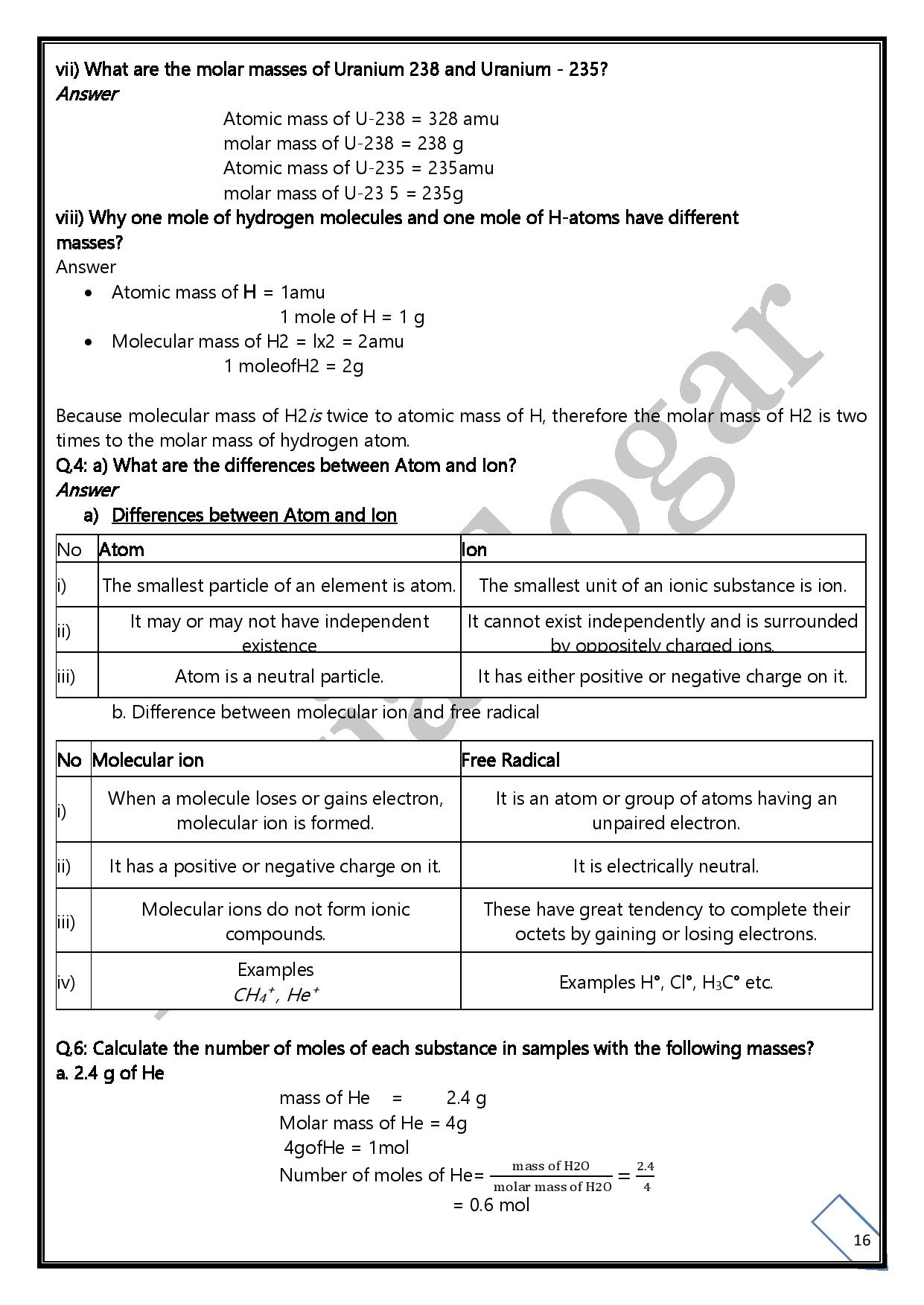Q.6: Calculate the number of moles of each substance in samples with the following masses?

1. 2.4 g of He

mass of He             =              2.4 g

Molar mass of He = 4g

4gofHe = 1mol

Number of moles of He= 2.4/4

= 0.6 mol

1. 250 mg of Carbon

Molar mass of carbon = 12 g

Mass of carbon        = 250 mg            = 250/1000 = 0.25 g

Number of moles =0.25/12 m=         0.02 mol

1. 15g of Sodium Chloride

Molar mass of Sodium Chloride =     23 + 35.5

=    58.5g

Mass of NaCl                         =              15/58.5

=              0.25 mol

1. 40 g Sulphur

Mass of sulphur                    =              40 g

Molar mass of S                    =              32 g

Number of moles =              =              40/32

=              1.25 mol

1. 5 Kg of MgO

Mass of MgO                         = 1.5 Kg

=1.5×1000 = 1500 g

Molar mass of MgO             =              40 g

Number of moles                 =              1500/40

=              3.5 mol

Q.7: Calculate the mass in grams of the following samples?

1. 2 moles of K

Molar mass of K = 39g

Number of moles= 1.2 mol

Mass of K = 39x 1.2 = 46.8g

1. 75 moles of H2

Molar mass of H2 = 2g

Moles of H2                          = 75 mol

Mass of H2                   = 2×75 = 150g

1. 0.25 moles of Steam

Molar mass of Steam(H20) = 2 + 16 = 18g

mol of steam = 0.25 mol

mass of steam       = 0.25x 18

=4.5g

1. 05 moles of CuSO4.5H2O

Molar mass of CuSO4.5H2O=

= 64 + 32 + 4(16)+ 5 (18)

= 64 + 32 + 64 + 90 = 249.5.g/mol

Moles of CuSO4.5H2O          = 1.05 mol

Mass of CuSO4.5H2O            =250 x 1.05 = 262.5g

1. 15 moles of H2S04

Molar mass of H2SO4 =        2 + 32 + 64

=              98g

Number of moles of H2SO=              0.15 mol

Mass of H2SO4                                                           = 98×0.15= 14.7gQ.8: Calculate the number of molecules present in each of the following samples?

1. 5 moles of Carbon dioxide

1 mole of CO2 =    6.02 x 1023 molecules

2.5 moles of C02 =                6.02x 1023x2.5

= 1.5×1024 molecules

1. 4 moles of ammonia, NH3

1 mole of NH3 = 6.02 x 1023 molecules

• moles ofNH3 = 6.02x 1023x 3.4 = 2 x 1024
1. 09 moles of benzene, C6H6

1 mol ofC6H6               =             6.02 x 1023 molecules

1.09 mol of C6H6     =             6.02 x 1023x 1.09

=              6.56 x 1023 molecules

1. 01 moles of acetic acid, CH3COOH

1 molofCH3COOH = 6.02 x 1023 molecules

0.01 molofCH3COOH = 6.02 x 1023x 0.01

= 6.0 x 1021 molecules

Q.9: Decide whether or not each of the following is an example of empirical formula:

1. a) Al2CI6b) Hg2Cl2 c)NaCl d) C2H6O
2. A12CI6

It is a molecular formula. Its empirical formula is AICI3.

1. Hg2Cl2

It is molecular formula &Empirical Formula = HgCl

1. NaCl

Yes. It is example of an empirical formula.

1. C6H6O

Yes. It is example of an empirical formula.

Q.10: TNT or trinitrotoluene is an explosive compound used in bombs. It contains 7 C-atoms, 6 H-atoms, 5 N-atoms and 6 O-atoms. Write its empirical formula?

Molecular formula of TNT = C7H6N5O6

Empirical formula of TNT = C7H6N506

Q.11: A molecule contains four phosphorus atoms and ten oxygen atoms. Write the empirical formula of this compound. Also determine the molar mass of this molecule?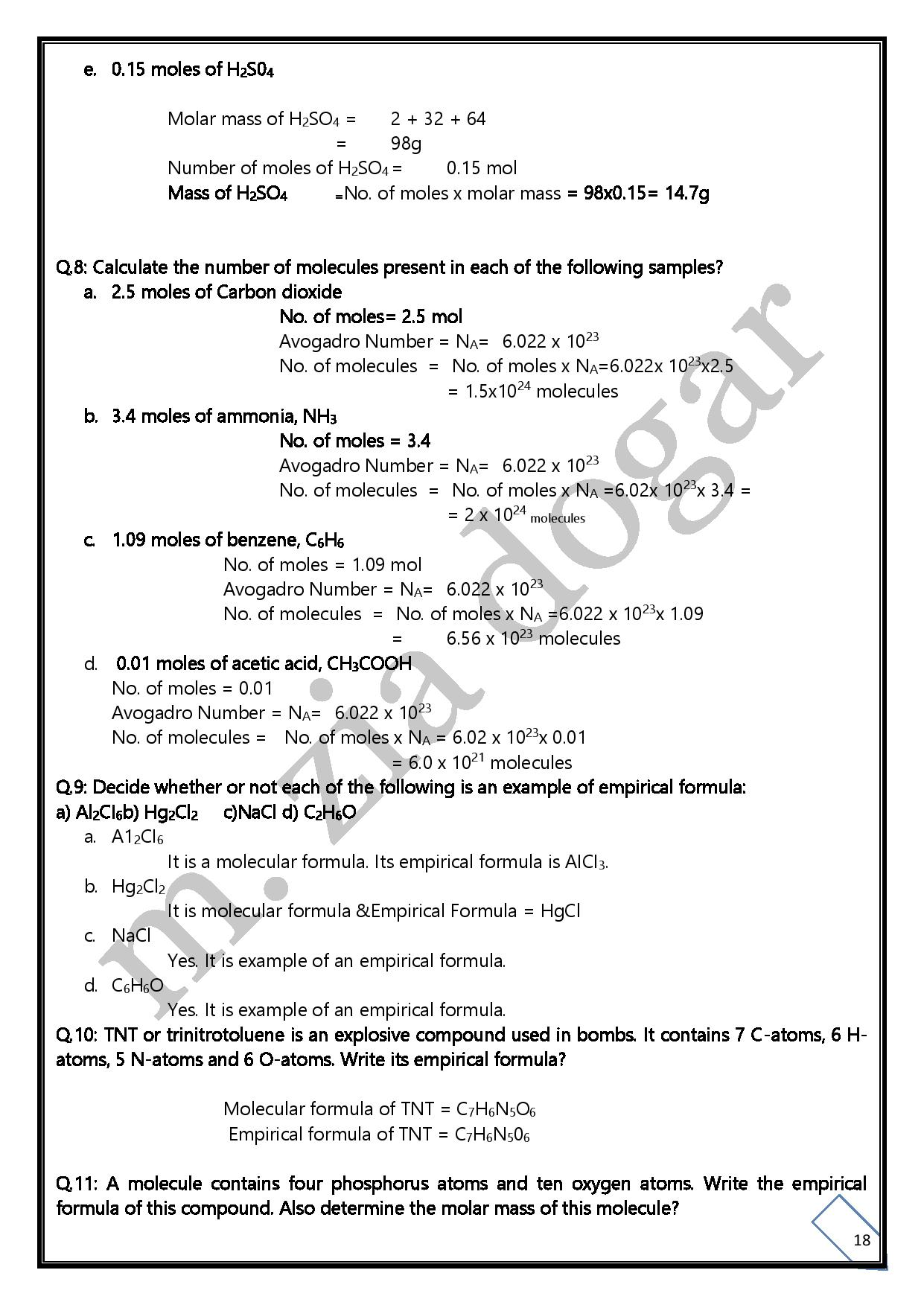Q.16: Identify the branch of chemistry that deals with the following examples:

• A cornstalk grows from a seed.
• Dynamite (C3H5N3O9) explodes to form a mixture of gases.
• Purple iodine vapour appears when solid iodine is warmed.
• Gasoline (a mixture of hydrocarbons) fumes are ignited in an auto mobile engine.
• A silver article tarnishes in air.
• Ice floats on water.
• Sulphur dioxide is the major source of acid rain.
• Many other light chlorinated hydrocarbons in drinking water are carcinogens.
• In Pakistan most of the factories use wet process for the production of cement.
• Carbon-14 is continuously produced in the atmosphere when high energy neutrons from space collide with nitrogen-14.

1. Biochemistry
2. Analytical chemistry
3. Environmental chemistry
4. Environmental chemistry
5. Industrial chemistry
6. Inorganic chemistry
7. Organic chemistry
8. Physical chemistry
9. Environmental chemistry
10. Nuclear chemistry